This tutorial focuses on using pandas to query experiment metadata for intracellular electrophysiology experiments using the metadata tables from the `icephys` module. See the Intracellular Electrophysiology tutorial for an introduction to the intracellular electrophysiology metadata tables and how to create an NWBFile for intracellular electrophysiology data.

Note

To enhance display of large pandas DataFrames, we save and render large tables as images in this tutorial. Simply click on the rendered table to view the full-size image.

## Imports used in the tutorial¶

```import os
```

Settings for improving rendering of tables in the online tutorial

```import dataframe_image

# Standard Python imports
import numpy as np
import pandas

# Get the path to the this tutorial
try:
tutorial_path = os.path.abspath(__file__)  # when running as a .py
except NameError:
tutorial_path = os.path.abspath("__file__")  # when running as a script or notebook
# directory to save rendered dataframe images for display
df_basedir = os.path.abspath(
os.path.join(
os.path.dirname(tutorial_path), "../../source/tutorials/domain/images/"
)
)
# Create the image directory. This is necessary only for gallery tests on GitHub
# but not for normal doc builds the output path already exists
os.makedirs(df_basedir, exist_ok=True)
# Set rendering options for tables
pandas.set_option("display.max_colwidth", 30)
pandas.set_option("display.max_rows", 10)
pandas.set_option("display.max_columns", 6)
dfi_fontsize = 7  # Fontsize to use when rendering with dataframe_image
```

## Example setup¶

Generate a simple example NWBFile with dummy intracellular electrophysiology data. This example uses a utility function `create_icephys_testfile` to create a dummy NWB file with random icephys data.

```from pynwb.testing.icephys_testutils import create_icephys_testfile

test_filename = "icephys_pandas_testfile.nwb"
nwbfile = create_icephys_testfile(
filename=test_filename,  # Write the file to disk for testing
randomize_data=True,  # Randomize the data in the simulus and response
with_missing_stimulus=True,  # Don't include the stimulus for row 0 and 10
)
```

## Accessing the ICEphys metadata tables¶

### Get the parent metadata table¶

The intracellular electrophysiology metadata consists of a hierarchy of DynamicTables, i.e., `ExperimentalConditionsTable` –> `RepetitionsTable` –> `SequentialRecordingsTable` –> `SimultaneousRecordingsTable` –> `IntracellularRecordingsTable`. However, in a given `NWBFile`, not all tables may exist - a user may choose to exclude tables from the top of the hierarchy (e.g., a file may only contain `SimultaneousRecordingsTable` and `IntracellularRecordingsTable` while omitting all of the other tables that are higher in the hierarchy). To provide a consistent interface for users, PyNWB allows us to easily locate the table that defines the root of the table hierarchy via the function `get_icephys_meta_parent_table`.

```root_table = nwbfile.get_icephys_meta_parent_table()
print(root_table.neurodata_type)
```
```ExperimentalConditionsTable
```

### Getting a specific ICEphys metadata table¶

We can retrieve any of the ICEphys metadata tables via the corresponding properties of NWBFile, i.e., `intracellular_recordings`, `icephys_simultaneous_recordings`, `icephys_sequential_recordings`, `icephys_repetitions`, `icephys_experimental_conditions`. The property will be `None` if the file does not contain the corresponding table. As such we can also easily check if a NWBFile contains a particular ICEphys metadata table via, e.g.:

```nwbfile.icephys_sequential_recordings is not None
```
```True
```

Warning

Always use the `NWBFile` properties rather than the corresponding get methods if you only want to retrieve the ICEphys metadata tables. The get methods (e.g., `get_icephys_simultaneous_recordings`) are designed to always return a corresponding ICEphys metadata table for the file and will automatically add the missing table (and all required tables that are lower in the hierarchy) to the file. This behavior is to ease populating the ICEphys metadata tables when creating or updating an `NWBFile`.

### Inspecting the table hierarchy¶

For any given table we can further check if and which columns are foreign `DynamicTableRegion` columns pointing to other tables via the the `has_foreign_columns` and `get_foreign_columns`, respectively.

```print("Has Foreign Columns:", root_table.has_foreign_columns())
print("Foreign Columns:", root_table.get_foreign_columns())
```
```Has Foreign Columns: True
Foreign Columns: ['repetitions']
```

Using `get_linked_tables` we can then also look at all links defined directly or indirectly from a given table to other tables. The result is a `list` of `typing.NamedTuple` objects containing, for each found link, the:

```linked_tables = root_table.get_linked_tables()

print(
"%s (%s, %s) ----> %s"
% (
"    " * i,
)
)
```
```(experimental_conditions, repetitions) ----> repetitions
(repetitions, sequential_recordings) ----> sequential_recordings
(sequential_recordings, simultaneous_recordings) ----> simultaneous_recordings
(simultaneous_recordings, recordings) ----> intracellular_recordings
```

## Converting ICEphys metadata tables to pandas DataFrames¶

### Using nested DataFrames¶

Using the `to_dataframe` method we can easily convert tables to pandas DataFrames.

```exp_cond_df = root_table.to_dataframe()
exp_cond_df
```
repetitions temperature
id
100000 sequential_... 32.0
100001 sequential_... 24.0

By default, the method will resolve `DynamicTableRegion` references and include the rows that are referenced in related tables as DataFrame objects, resulting in a hierarchically nested DataFrame. For example, looking at a single cell of the `repetitions` column of our `ExperimentalConditionsTable` table, we get the corresponding subset of repetitions from the py:class:~pynwb.icephys.RepetitionsTable.

```exp_cond_df.iloc["repetitions"]
```
sequential_recordings type
id
10000 simultaneous_r... R1
10001 simultaneous_r... R2

In contrast to the other ICEphys metadata tables, the `IntracellularRecordingsTable` does not contain any `DynamicTableRegion` columns, but it is a `AlignedDynamicTable` which contains sub-tables for `electrodes`, `stimuli`, and `responses`. For convenience, the `to_dataframe` of the `IntracellularRecordingsTable` provides a few additional optional parameters to ignore the ids of the category tables (via `ignore_category_ids=True`) or to convert the electrode, stimulus, and response references to ObjectIds. For example:

```ir_df = nwbfile.intracellular_recordings.to_dataframe(
ignore_category_ids=True,
electrode_refs_as_objectids=True,
stimulus_refs_as_objectids=True,
response_refs_as_objectids=True,
)

# save the table as image to display in the docs
dataframe_image.export(
obj=ir_df,
filename=os.path.join(df_basedir, "intracellular_recordings_dataframe.png"),
table_conversion="matplotlib",
fontsize=dfi_fontsize,
)
```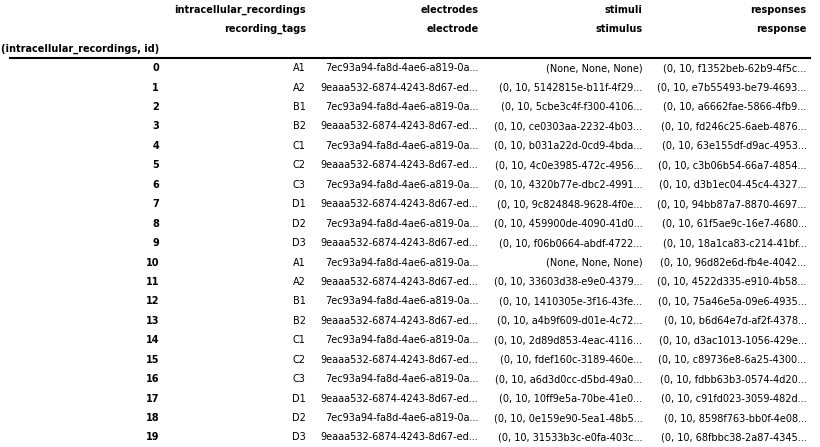### Using indexed DataFrames¶

Depending on the particular analysis, we may be interested only in a particular table and do not want to recursively load and resolve all the linked tables. By setting `index=True` when converting the table `to_dataframe` the `DynamicTableRegion` links will be represented as lists of integers indicating the rows in the target table (without loading data from the referenced table).

```root_table.to_dataframe(index=True)
```
repetitions temperature
id
100000 [0, 1] 32.0
100001 [2, 3] 24.0

To resolve links related to a set of rows, we can then simply use the corresponding `DynamicTableRegion` column from our original table, e.g.:

```root_table["repetitions"][
0
]  # Look-up the repetitions for the first experimental condition
```
sequential_recordings type
id
10000 simultaneous_r... R1
10001 simultaneous_r... R2

We can also naturally resolve links ourselves by looking up the relevant table and then accessing elements of the table directly.

```# All DynamicTableRegion columns in the ICEphys table are indexed so we first need to
# follow the ".target" to the VectorData and then look up the table via ".table"
target_table = root_table["repetitions"].target.table
target_table[[0, 1]]
```
sequential_recordings type
id
10000  R1
10001 [1, 2] R2

Note

We can also explicitly exclude the `DynamicTableRegion` columns (or any other column) from the DataFrame using e.g., `root_table.to_dataframe(exclude={'repetitions', })`.

### Using a single, hierarchical DataFrame¶

To gain a more direct overview of all metadata at once and avoid iterating across levels of nested DataFrames during analysis, it can be useful to flatten (or unnest) nested DataFrames, expanding the nested DataFrames by adding their columns to the main table, and expanding the corresponding rows in the parent table by duplicating the data from the existing columns across the new rows. For example, an experimental condition represented by a single row in the `ExperimentalConditionsTable` containing 5 repetitions would be expanded to 5 rows, each containing a copy of the metadata from the experimental condition along with the metadata of one of the repetitions. Repeating this process recursively, a single row in the `ExperimentalConditionsTable` will then ultimately expand to the total number of intracellular recordings from the `IntracellularRecordingsTable` that belong to the experimental conditions table.

HDMF povides several convenience functions to help with this process. Using the `to_hierarchical_dataframe` method, we can transform our hierarchical table into a single pandas DataFrame. To avoid duplication of data in the display, the hierarchy is represented as a pandas MultiIndex on the rows so that only the data from the last table in our hierarchy (i.e. here the `IntracellularRecordingsTable`) is represented as columns.

```from hdmf.common.hierarchicaltable import to_hierarchical_dataframe

icephys_meta_df = to_hierarchical_dataframe(root_table)

# save table as image to display in the docs
dataframe_image.export(
obj=icephys_meta_df,
filename=os.path.join(df_basedir, "icephys_meta_dataframe.png"),
table_conversion="matplotlib",
fontsize=dfi_fontsize,
)
```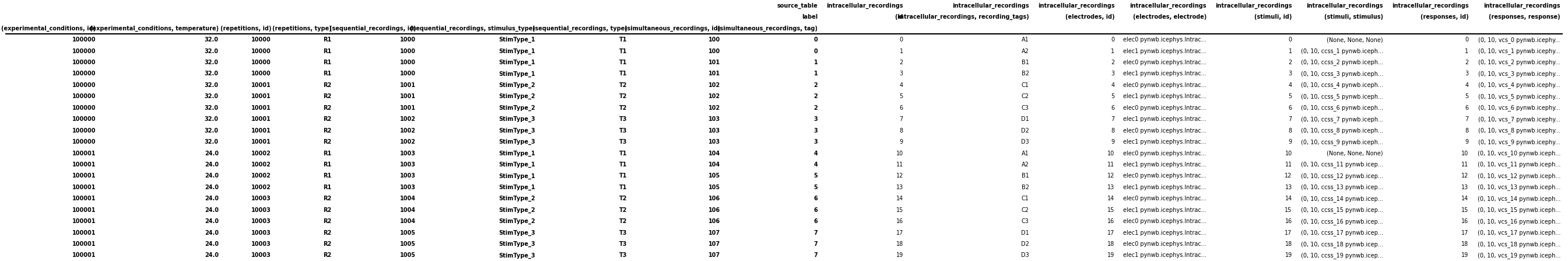Depending on the analysis, it can be useful to further process our DataFrame. Using the standard reset_index function, we can turn the data from the MultiIndex to columns of the table itself, effectively denormalizing the display by repeating all data across rows. HDMF then also provides: 1) `drop_id_columns` to remove all “id” columns and 2) `flatten_column_index` to turn the MultiIndex on the columns of the table into a regular Index of tuples.

Note

Dropping `id` columns is often useful for visualization purposes while for query and analysis it is often useful to maintain the `id` columns to facilitate lookups and correlation of information.

```from hdmf.common.hierarchicaltable import drop_id_columns, flatten_column_index

# Reset the index of the dataframe and turn the values into columns instead
icephys_meta_df.reset_index(inplace=True)
# Flatten the column-index, turning the pandas.MultiIndex into a pandas.Index of tuples
flatten_column_index(dataframe=icephys_meta_df, max_levels=2, inplace=True)
# Remove the id columns. By setting inplace=False allows us to visualize the result of this
# action while keeping the id columns in our main icephys_meta_df table
drid_icephys_meta_df = drop_id_columns(dataframe=icephys_meta_df, inplace=False)

# save the table as image to display in the docs
dataframe_image.export(
obj=drid_icephys_meta_df,
filename=os.path.join(df_basedir, "icephys_meta_dataframe_drop_id.png"),
table_conversion="matplotlib",
fontsize=dfi_fontsize,
)
```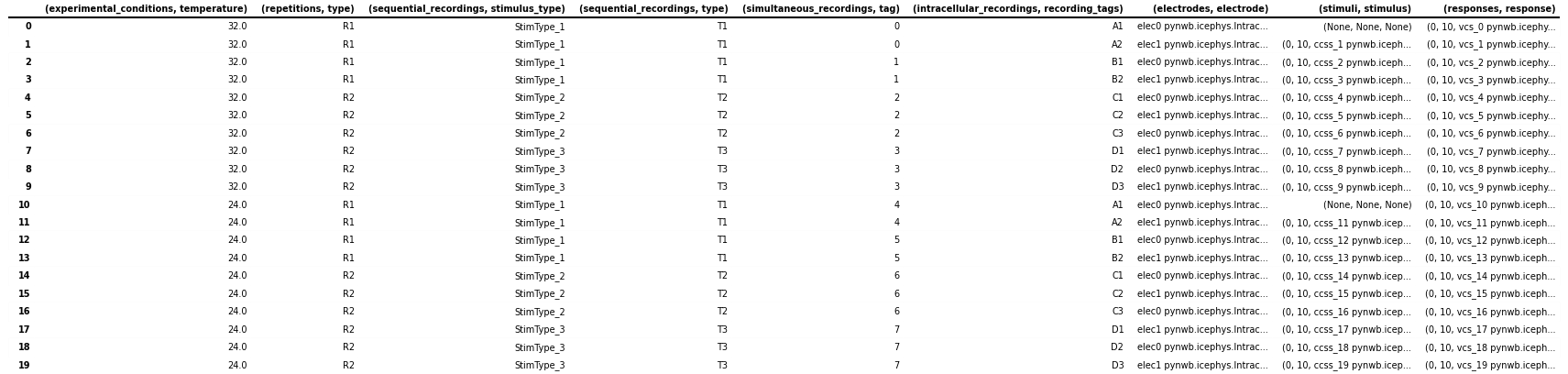### Expanding TimeSeriesReference columns¶

For query purposes it can be useful to expand the stimulus and response columns to separate the `(start, count, timeseries)` values in separate columns. This is primarily useful if we want to perform queries on these components directly, otherwise it is usually best to keep the stimulus/response references around as :py:class:`~pynwb.base.TimeSeriesReference, which provides additional features to inspect and validate the references and load data. We, therefore, here keep the data in both forms in the table

```# Expand the ('stimuli', 'stimulus') to a DataFrame with 3 columns
stimulus_df = pandas.DataFrame(
icephys_meta_df[("stimuli", "stimulus")].tolist(),
columns=[("stimuli", "idx_start"), ("stimuli", "count"), ("stimuli", "timeseries")],
index=icephys_meta_df.index,
)
# If we want to remove the original ('stimuli', 'stimulus') from the dataframe we can call
# icephys_meta_df.drop(labels=[('stimuli', 'stimulus'), ], axis=1, inplace=True)
# Add our expanded columns to the icephys_meta_df dataframe
icephys_meta_df = pandas.concat([icephys_meta_df, stimulus_df], axis=1)

# save the table as image to display in the docs
dataframe_image.export(
obj=icephys_meta_df,
filename=os.path.join(df_basedir, "icephys_meta_dataframe_expand_tsr.png"),
table_conversion="matplotlib",
fontsize=dfi_fontsize,
)
```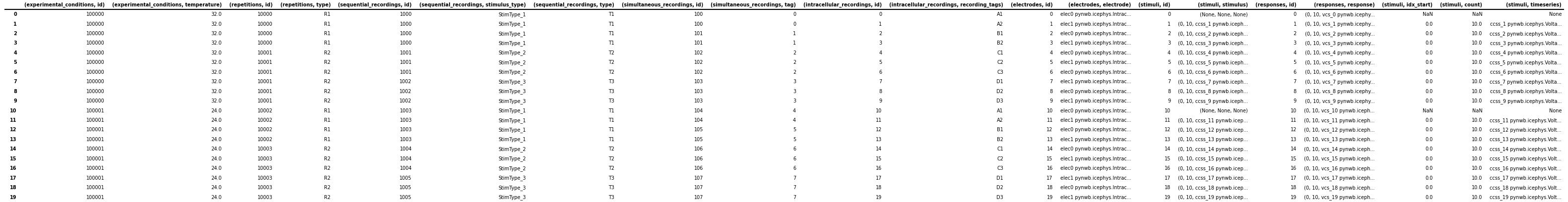We can then easily expand also the `(responses, response)` column in the same way

```response_df = pandas.DataFrame(
icephys_meta_df[("responses", "response")].tolist(),
columns=[
("responses", "idx_start"),
("responses", "count"),
("responses", "timeseries"),
],
index=icephys_meta_df.index,
)
icephys_meta_df = pandas.concat([icephys_meta_df, response_df], axis=1)
```

With all TimeSeries stimuli and responses listed in the table, we can easily iterate over the TimeSeries to expand our table with additional columns with information from the TimeSeries, e.g., the `neurodata_type` or `name` or any other properties we may wish to extract from our stimulus and response TimeSeries (e.g., `rate`, `starting_time`, `gain` etc.). Here we show a few examples.

```# Add a column with the name of the stimulus TimeSeries object.
# Note: We use getattr here to easily deal with missing values,
#       i.e., here the cases where no stimulus is present
col = ("stimuli", "name")
icephys_meta_df[col] = [
getattr(s, "name", None) for s in icephys_meta_df[("stimuli", "timeseries")]
]

# Often we can easily do this in a bulk-fashion by specifying
# the collection of fields of interest
for field in ["neurodata_type", "gain", "rate", "starting_time", "object_id"]:
col = ("stimuli", field)
icephys_meta_df[col] = [
getattr(s, field, None) for s in icephys_meta_df[("stimuli", "timeseries")]
]
# save the table as image to display in the docs
dataframe_image.export(
obj=icephys_meta_df,
table_conversion="matplotlib",
max_cols=10,
fontsize=dfi_fontsize,
)
```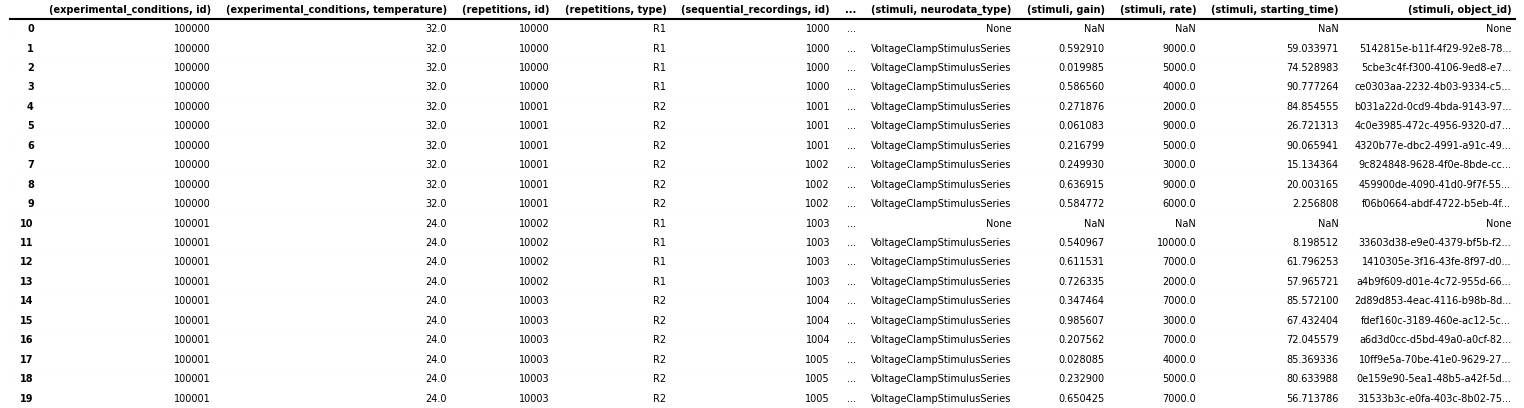Naturally we can again do the same also for our response columns

```for field in ["name", "neurodata_type", "gain", "rate", "starting_time", "object_id"]:
col = ("responses", field)
icephys_meta_df[col] = [
getattr(s, field, None) for s in icephys_meta_df[("responses", "timeseries")]
]
```

And we can use the same process to also gather additional metadata about the `IntracellularElectrode`, `Device` and others

```for field in ["name", "device", "object_id"]:
col = ("electrodes", field)
icephys_meta_df[col] = [
getattr(s, field, None) for s in icephys_meta_df[("electrodes", "electrode")]
]
```

This basic approach allows us to easily collect all data needed for query in a convenient spreadsheet for display, query, and analysis.

With regard to the experiment metadata tables, many of the queries we identified based on feedback from the community follow the model of: “Given X return Y”, e.g.:

• Given a particular stimulus return:
• the corresponding response

• the corresponding electrode

• the stimulus type

• all stimuli/responses recorded at the same time (i.e., during the same simultaneous recording)

• all stimuli/responses recorded during the same sequential recording

• Given a particular response return:
• the corresponding stimulus

• the corresponding electrode

• all stimuli/responses recorded at the same time (i.e., during the same simultaneous recording)

• all stimuli/responses recorded during the same sequential recording

• Given an electrode return:
• all responses (and stimuli) related to the electrode

• all sequential recordings (a.k.a., sweeps) recorded with the electrode

• Given a stimulus type return:
• all related stimulus/response recordings

• all the repetitions in which it is present

• Given a stimulus type and a repetition return:
• all the responses

• Given a simultaneous recording (a.k.a., sweep) return:
• the repetition/condition/sequential recording it belongs to

• all other simultaneous recordings that are part of the same repetition

• the experimental condition the simultaneous recording is part of

• Given a repetition return:
• the experimental condition the simultaneous recording is part of

• all sequential- and/or simultaneous recordings within that repetition

• Given an experimental condition return:
• All corresponding repetitions or sequential/simultaneous/intracellular recordings

• Get the list of all stimulus types

More complex analytics will then commonly combine multiple such query constraints to further process the corresponding data, e.g.,

• Given a stimulus and a condition, return all simultaneous recordings (a.k.a., sweeps) across repetitions and average the responses

Generally, many of the queries involve looking up a piece of information in on table (e.g., finding a stimulus type in `SequentialRecordingsTable`) and then querying for related information in child tables (by following the `DynamicTableRegion` links included in the corresponding rows) to look up more specific information (e.g., all recordings related to the stimulus type) or alternatively querying for related information in parent tables (by finding rows in the parent table that link to our rows) and then looking up more general information (e.g., information about the experimental condition). Using this approach, we can resolve the above queries using the individual `DynamicTable` objects directly, while loading only the data that is absolutely necessary into memory.

With the bulk data stored usually in some form of `PatchClampSeries`, the ICEphys metadata tables will usually be comparatively small (in terms of total memory). Once we have created our integrated DataFrame as shown above, performing the queries described above becomes quite simple as all links between tables have already been resolved and all data has been expanded across all rows. In general, resolving queries on our “denormalized” table amounts to evaluating one or more conditions on one or more columns and then retrieving the rows that match our conditions form the table.

Once we have all metadata in a single table, we can also easily sort the rows of our table based on a flexible set of conditions or even cluster rows to compute more advanced groupings of intracellular recordings.

Below we show just a few simple examples:

### Given a response, get the stimulus¶

```# Get a response 'vcs_9' from the file
response = nwbfile.get_acquisition("vcs_9")
# Return all data related to that response, including the stimulus
# as part of ('stimuli', 'stimulus') column
icephys_meta_df[icephys_meta_df[("responses", "object_id")] == response.object_id]
```
(experimental_conditions, id) (experimental_conditions, temperature) (repetitions, id) ... (electrodes, name) (electrodes, device) (electrodes, object_id)
9 100000 32.0 10001 ... elec1 Heka ITC-1600 pynwb.device... 90a6bd34-7166-41d3-8368-34...

1 rows × 38 columns

### Given a response load the associated data¶

References to timeseries are stored in the `IntracellularRecordingsTable` via `TimeSeriesReferenceVectorData` columns which return the references to the stimulus/response via `TimeSeriesReference` objects. Using `TimeSeriesReference` we can easily inspect the selected data.

```ref = icephys_meta_df[("responses", "response")]  # Get the TimeSeriesReference
_ = ref.isvalid()  # Is the reference valid
_ = ref.idx_start  # Get the start index
_ = ref.count  # Get the count
_ = ref.timeseries.name  # Get the timeseries
_ = ref.timestamps  # Get the selected timestamps
ref_data = ref.data  # Get the selected recorded response data values
# Print the data values just as an example
print("data = " + str(ref_data))
```
```data = [0.53490464 0.1517095  0.05106839 0.00673373 0.98138589 0.66615288
0.60875091 0.68105936 0.41034665 0.82962513]
```

### Get a list of all stimulus types¶

```unique_stimulus_types = np.unique(
icephys_meta_df[("sequential_recordings", "stimulus_type")]
)
print(unique_stimulus_types)
```
```['StimType_1' 'StimType_2' 'StimType_3']
```

### Given a stimulus type, get all corresponding intracellular recordings¶

```query_res_df = icephys_meta_df[
icephys_meta_df[("sequential_recordings", "stimulus_type")] == "StimType_1"
]

# save the table as image to display in the docs
dataframe_image.export(
obj=query_res_df,
filename=os.path.join(df_basedir, "icephys_meta_query_result_dataframe.png"),
table_conversion="matplotlib",
max_cols=10,
fontsize=dfi_fontsize,
)
```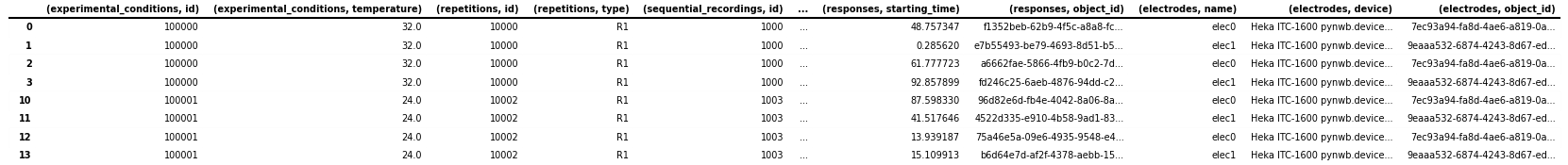Gallery generated by Sphinx-Gallery